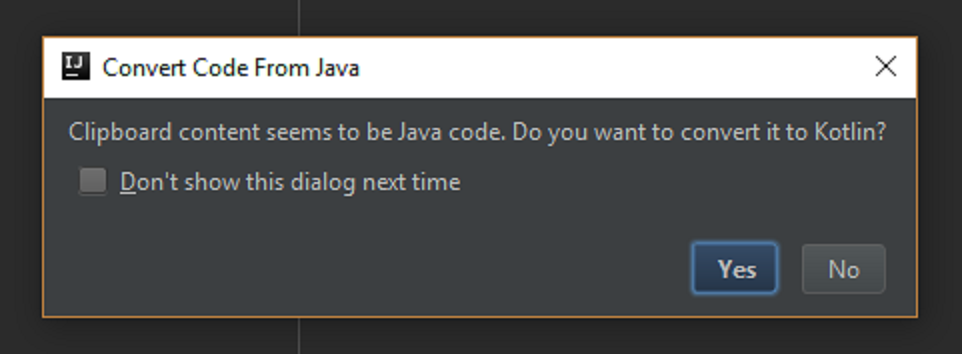# 你应该切换到Kotlin开发

Kotlin是JetBrains公司开发的。JetBrains实际上是开发IDES。例如IntelliJReSharper。真正闪耀则是通过Kotlin,Kotlin是简洁和务实的,并且使得编码成为了令人满意和有效的体验。

### 一、与Java的互通性

Kotlin能够达到和JAVA的100%互通,也就是说你可以使用Kotlin开发您的旧的JAVA项目.所有的你喜欢的Java框架都可以继续使用.而且你写的一些Kotlin的框架也都能非常容易的服务于你的java朋友爱好者.

### 二、熟悉的语法

Kotlin不是学术界(编程界)奇怪的(令人难以捉摸的语言).来自于OOP(面向对象编程语言)界的程序员都很熟悉.并且或多或少的都可以去理解.当然与Java还是有一定的区别的.比如说构造函数,或者是val,var变量声明.(下面的代码段则提供了一部分基础的说明)

``````class Foo {

val b: String = "b"     // val means unmodifiable  不可以修改
var i: Int = 0          // var means modifiable  可以修改

fun hello() {
val str = "Hello"
print("\$str World")
}

fun sum(x: Int, y: Int): Int {
return x + y
}

fun maxOf(a: Float, b: Float) = if (a > b) a else b

}
``````

### 三、字符串差值

``````val x = 4
val y = 7
print("sum of \$x and \$y is \${x + y}")  // sum of 4 and 7 is 11
``````

### 四、类型推断

Kotlin会推断你的想要的类型(你将会觉得提高代码的阅读性)

``````val a = "abc"                         // type inferred to String
val b = 4                             // type inferred to Int

val c: Double = 0.7                   // type declared explicitly
val d: List<String> = ArrayList()     // type declared explicitly
``````

### 五、聪明的类型转换

Kotlin编译器会跟踪您的逻辑做自动转换类型,这意味着不会有过多的instanceof检测(在明确的转换)

``````if (obj is String) {
print(obj.toUpperCase())     // obj is now known to be a String
}
``````

### 六、直观上的相等

``````val john1 = Person("John")
val john2 = Person("John")

john1 == john2    // true  (structural equality)
john1 === john2   // false (referential equality)
``````

### 七、默认参数

``````fun build(title: String, width: Int = 800, height: Int = 600) {
Frame(title, width, height)
}
``````

### 八、命名参数

``````build("PacMan", 400, 300)                           // equivalent
build(title = "PacMan", width = 400, height = 300)  // equivalent
build(width = 400, height = 300, title = "PacMan")  // equivalent
``````

### 九、when表达式

switch语句被替换成更加易读和灵活的when表达式

``````when (x) {
1 -> print("x is 1")
2 -> print("x is 2")
3, 4 -> print("x is 3 or 4")
in 5..10 -> print("x is 5, 6, 7, 8, 9, or 10")
else -> print("x is out of range")
}
``````

``````val res: Boolean = when {
obj == null -> false
obj is String -> true
else -> throw IllegalStateException()
}
``````

### 十、属性

``````class Frame {
var width: Int = 800
var height: Int = 600

val pixels: Int
get() = width * height
}
``````

### 十一、数据类(类似JavaBean)

``````data class Person(val name: String,
var email: String,
var age: Int)

val john = Person("John", "john@gmail.com", 112)
``````

### 十二、运算符重载

``````data class Vec(val x: Float, val y: Float) {
operator fun plus(v: Vec) = Vec(x + v.x, y + v.y)
}

val v = Vec(2f, 3f) + Vec(4f, 1f)
``````

### 十三、解构说明(Destructuring Declarations)

``````for ((key, value) in map) {
print("Key: \$key")
print("Value: \$value")
}
``````

### 十四、范围(Ranges)

``````for (i in 1..100) { ... }
for (i in 0 until 100) { ... }
for (i in 2..10 step 2) { ... }
for (i in 10 downTo 1) { ... }
if (x in 1..10) { ... }
``````

### 十五、扩展方法/功能

``````fun String.format(): String {
return this.replace(' ', '_')
}

val formatted = str.format()
``````

``````str.removeSuffix(".txt")
str.capitalize()
str.substringAfterLast("/")
str.replaceAfter(":", "classified")
``````

### 十六、空值安全

Java是一种应该称之为几乎静态类型的语言,在Java中,String类型的变量不能保证引用String(安全) – 它可能引用为null,即使我们已经习惯了这个类型,它抵消了静态类型检查的安全性,所以Java开发人员将不会避免的遇见NPEs.(NullPointerException)或者想法设法的避免(有点多于的工作)
Kotlin则是通过区分非空类型和空类型来解决这个问题。默认情况下，类型为非空，并且可以默认添加一个？例如以下这样:

``````var a: String = "abc"
a = null                // compile error

var b: String? = "xyz"
b = null                // no problem
``````

``````val x = b.length        // compile error: b might be null
``````

``````if (b == null) return
val x = b.length        // no problem
``````

``````val x = b?.length       // type of x is nullable Int
``````

``````val name = ship?.captain?.name ?: "unknown"
``````

``````val x = b?.length ?: throw NullPointerException()  // same as below
val x = b!!.length                                 // same as above
``````

### 十七、更好的Lambda表达式

``````val sum = { x: Int, y: Int -> x + y }   // type: (Int, Int) -> Int
val res = sum(4,7)                      // res == 11
``````

• 1、如果lambda表达式的是方法的最后一个参数或唯一的参数，则可以移动或省略方法括号
• 2、如果我们选择不声明一个single-argument-lambda，它将被隐式声明为`it`
由以上两点可以得到以下三行代码形式：
``````numbers.filter({ x -> x.isPrime() })
numbers.filter { x -> x.isPrime() }
numbers.filter { it.isPrime() }
``````

``````persons
.filter { it.age >= 18 }
.sortedBy { it.name }
.map { it.email }
.forEach { print(it) }
``````

Kotlin的lambda系统功能的完美结合使其成为DSL创建的理想选择,查看Anko的DSL示例，旨在服务Android开发.

``````verticalLayout {
editText {
hint = “Name”
textSize = 24f
}
editText {
textSize = 24f
}
textSize = 26f
}
}
``````

### 十八、IDE支持IntelliJ will notice if you paste Java code into a Kotlin file

```作者：若兰明月